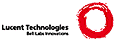Plan 9 from Bell Labs’s /usr/web/sources/contrib/de0u/blame

 ``` #!/bin/rc # Dave Eckhardt, 2009-04-12 rfork ne fn usage { msg='usage: '^`{basename \$0}^' [-b] executable-file [object-file|library...]' echo \$msg echo ' -b includes BSS (present in memory but absent from executable file)' echo ' -f report blame by file, not by symbol (you must provide objs/libs)' exit \$msg } fn missing { msg=\$1^': not found'; echo \$msg; exit \$msg } ~ \$#* 0 && usage # Plan # # Parse command line into flags, objects, libraries, and one executable. # Inventory objects and libraries into a symbol->origin hash. # For each executable symbol, compute size and origin, building blame hash. # Print blame hash. libs=() ; objs = () ; execf = () for (arg) { switch (\$arg) { case -b dobss=1 case -f blamefiles=1 case -* usage case *.a test -r \$arg || missing \$arg libs=(\$libs \$arg) case *.? *.a? test -r \$arg || missing \$arg objs=(\$objs \$arg) case * test -r \$arg || missing \$arg execf=(\$execf \$arg) } } # Exactly one executable file. ~ \$#execf 1 || usage # If we have only one intermediate file, nm will helpfully # omit to print the file name in its output. So double it. ~ \$#objs 1 && objs=(\$objs \$objs) BSSawk='BEGIN { dobss = 0 }' ~ \$#dobss 1 && BSSawk='BEGIN { dobss = 1}' BLAMEawk='BEGIN { blamefiles = 0 }' ~ \$#blamefiles 1 && BLAMEawk='BEGIN { blamefiles = 1 }' # Ok, enough meditation. Let's get to work. sizeoutput=(`{size \$execf}) size=\$sizeoutput(7) # We run nm manually multiple times so we can decorate # member names with the name of the library. Note that # we trim out symbol references here via grep to save awk # some work. Also, the order of these steps matters: the # executable must be last and sorted numerically. { for (lib in \$libs) { # Instead of saying that chatty9p comes from "thread.8", # rewrite to say it comes from "lib9p(thread.8)". b=`{basename \$lib | sed -e 's/\.a//'} nm \$lib | sed -e 's/(^[^:]*):/'^\$b'(\1):/' } ~ \$#objs 0 || nm \$objs nm -n \$execf } | grep -v ' U ' | \ \ awk 'BEGIN { totalsize = sprintf("%x",'^\$size^') }' ^ ' ' ^ \$"BSSawk ^ \$"BLAMEawk ^ ' BEGIN { for (i=0; i<16; i++) _unhex[sprintf("%x", i)] = _unhex[sprintf("%X", i)] = i got1origin = 0; } function unhex(s, i, v) { v = 0 for (i=1; i <= length(s); i++) v = v*16 + _unhex[substr(s,i,1)] return v } function originates(sym, file) { origin[sym] = file; got1origin = 1; } function entity(sym, start, end, kind, mysize, myfile) { # Ignore "small" things - ints in header files are "defined" multiply, but are only noise for our purposes if ((mysize = unhex(end) - unhex(start)) <= 64) return; if (!dobss && (kind == "b" || kind == "B")) return; if ((myfile = origin[sym]) == "") { myfile = "???" } if (blamefiles) { blame[myfile] += mysize } else { if (got1origin) print mysize, sym, myfile; else print mysize, sym; } } # First we expect to see lines like this: # xalloc.8: T xsummary # We key off the colon. ((\$1 ~ /^..*:\$/) && (NF == 3)) { originates(\$3, substr(\$1,1,length(\$1)-1)); } # Then we expect lines like this: # f0100020 T _startKADDR ((\$1 ~ /^[a-z0-9][a-z0-9]*\$/) && (NF == 3)) { if (!donefirst) { # "fill pipe" oldhex = \$1; oldkind = \$2; oldsymbol = \$3; donefirst = 1; } else { entity(oldsymbol, oldhex, \$1, oldkind); oldhex = \$1; oldkind = \$2; oldsymbol = \$3; } } END { # first flush last symbol from pipe entity(oldsymbol, oldhex, totalsize, oldkind); if (blamefiles) { for (file in blame) { print blame[file], file; } } } ' # awk code for unhex due to Russ Cox exit '' ```